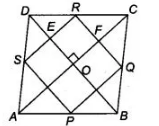# P, Q, R and S are respectively the mid-points of sides AB,`
Question:

P, Q, R and S are respectively the mid-points of sides AB, BC, CD and DA of quadrilateral ABCD in which AC = BD and AC ⊥ BD. Prove that PQRS is a

square.

Solution:

Given In quadrilateral ABCD, P, Q, R and S are the mid-points of the sides AB, BC, CD and DA, respectively.

Also, AC = BD and AC ⊥ BD.

To prove PQRS is a square.

Proof Now, in ΔADC, S and R are the mid-points of the sides AD and DC respectively, then by mid-point theorem,

$S R \| A C$ and $S R=\frac{1}{2} A C$ $\ldots($ i)In $\triangle A B C, P$ and $Q$ are the mid-points of $A B$ and $B C$, then by mid-point theorem,

$P Q \| A C$ and $P Q=\frac{1}{2} A C$ $\ldots$ (ii)

From Eqs. (i) and (ii), $P Q \| S R$ and $P Q=S R=\frac{1}{2} A C$ ....(iii)

Similarly, in $\triangle A B D$, by mid-point theorem,

$S P \| B D$ and $S P=\frac{1}{2} B D=\frac{1}{2} A C \quad$ [given, $\left.A C=B D\right] \ldots$ (iv)

and $\triangle B C D$, by mid-point theorem,

$R Q \| B D$ and $R Q=\frac{1}{2} B D=\frac{1}{2} A C$  [given, $B D=A C$ ] ...(v)

From Eqs. (iv) and (v).

$S P=R Q=\frac{1}{2} A C$  ....(vi)

From Eqs. (iii) and (vi),

$P Q=S R=S P=R Q$

Thus, all four sides are equal.

Now, in quadrilateral $O E R F, \quad O E \| F R$ and $O F \| E R$

$\therefore \quad \angle E O F=\angle E R F=90^{\circ}$

$\left[\because A C \perp D B \Rightarrow=\angle D O C=\angle E O F=90^{\circ}\right.$ as opposite angles of a parallelogram $]$

$\angle Q R S=90^{\circ}$

Similarly,

$\angle R Q S=90^{\circ}$

So, $P Q R S$ is a square.

Hence proved.